Shell ScriptingUnderstanding Test Conditions in Linux Shell Scripting

# Understanding Test Conditions in Linux Shell Scripting

In this article, we are going to tackle very important topic: the test conditions. Test conditions are very important when it comes to making decisions.

The test Command
The test command is used to check file types and compare values.

Syntax:

`test EXPRESSION`

Where EXPRESSION could be a Comparison, File test, String test, or Logical Operation whose result is either true or false.

A short alternative to the above syntax is the following:

`[ EXPRESSION ]	`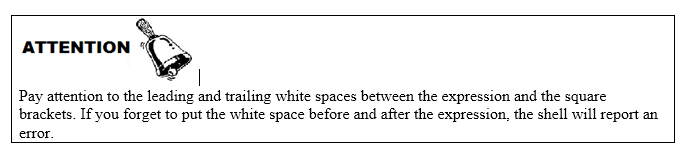EXPRESSION could be a simple condition, or a composite of two or more EXPRESSIONs. The conditions are operations which use operators that belong to either of the following types:

• Logical Operators.
• String Operators.
• Comparison Operators.
• File Test Operators.

The following table lists the operators of each type, with short description for each, and the syntax of usage:

 Operator Description Syntax Logical Operators ! Logical NOT. Inverts the result of the EXPRESSION ! EXPRESSION -a && Both EXPRESSION1 and EXPRESSION2 are true EXPRESSION1 –a EXPRESSION2 EXPRESSION1 && EXPRESSION2 -o || Either EXPRESSION1 or EXPRESSION2 is true EXPRESSION1 –o EXPRESSION2 EXPRESSION1 || EXPRESSION2 String Operators -n the length of STRING is nonzero -n STRING -z the length of STRING is zero -z STRING = the strings are equal STRING1 = STRING2 != the strings are not equal STRING1 != STRING2 Comparison Operators -eq INTEGER1 is equal to INTEGER2 INTEGER1 -eq INTEGER2 -ge INTEGER1 is greater than or equal to INTEGER2 INTEGER1 -ge INTEGER2 -gt INTEGER1 is greater than INTEGER2 INTEGER1 -gt INTEGER2 -le INTEGER1 is less than or equal to INTEGER2 INTEGER1 -le INTEGER2 -lt INTEGER1 is less than INTEGER2 INTEGER1 -lt INTEGER2 -ne INTEGER1 is not equal to INTEGER2 INTEGER1 -ne INTEGER2 File Test Operators -ef FILE1 and FILE2 have the same device and inode numbers FILE1 -ef FILE2 -nt FILE1 is newer (modification date) than FILE2 FILE1 -nt FILE2 -ot FILE1 is older than FILE2 FILE1 -ot FILE2 -b FILE exists and is block special -b FILE -c FILE exists and is character special -c FILE -d FILE exists and is a directory -d FILE -e FILE exists -e FILE -f FILE exists and is a regular file -f FILE -h FILE exists and is a symbolic link (same as -L) -h FILE -L FILE exists and is a symbolic link (same as -h) -L FILE -r FILE exists and read permission is granted -r FILE -s FILE exists and has a size greater than zero -s FILE -w FILE exists and write permission is granted -w FILE -x FILE exists and execute (or search) permission is granted -x FILE

Examples
The following examples shows the test conditions using the string operators.
Given that str=”Shell Scripting” and str2=”” (empty string):

• The following checks if the length of string variable str is non-zero (Not empty string):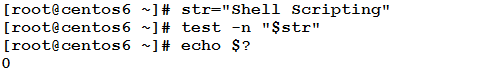Note that exit status of the test command is 0, which means the test was successful (true).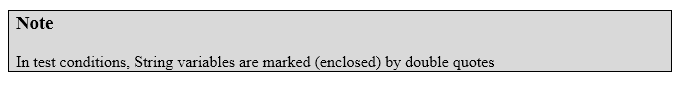• The following tests if the variable str is an empty string.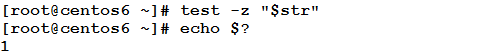The non-zero exit status means the test condition was unsuccessful (false).

• The following tests if str2 is an empty string: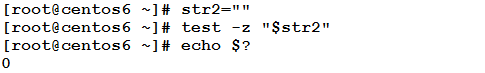The zero exit status means the test condition was true (str2 is really an empty string)

• The following test condition checks whether str equals str2.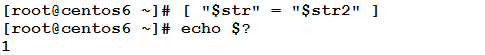• The following test statement checks whether str equal the literal string “Shell Scripting” (which is really the case, so it will evaluate to true giving 0 exit status)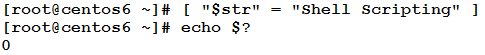• The following test statement checks whether str and str2 are not equal.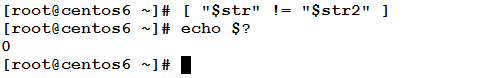Examples
In the following examples, we are going to see how Comparison Operators work.

Given x=5, y=z=8:

• The following test condition checks whether x is equal to y (which is not the case)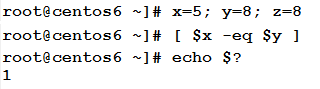• This also tests whether y equals z or not.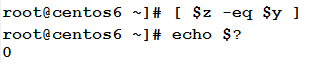• This test checks whether y is greater than or equal to x.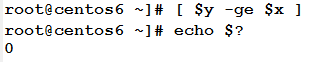• This test checks whether x is greater than z (which is false).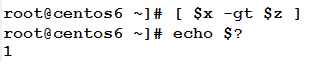• This test checks whether x is less than or equal to z (x is actually less than z, which makes the condition evaluates to true)• The following test checks whether y is less than x (which is not the case, as y is greater than x). The condition evaluates to false, as it appears in the non-zero exit status.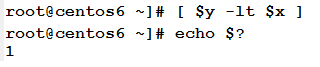• The following test checks whether y is not equal to z.Examples
The following are illustrative examples to show how File test operators work.

Given the following content in the current directory: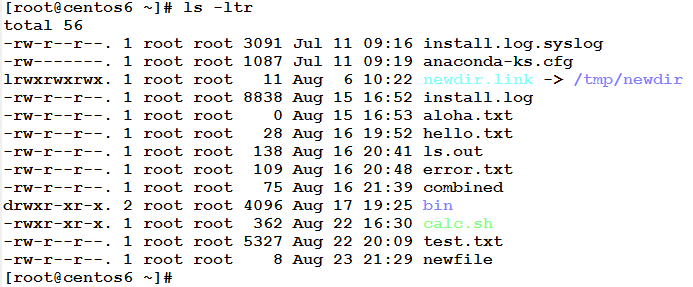• The following test condition checks if the file newfile is newer than test.txt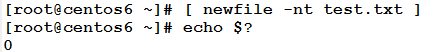The exit status of the test command indicates that condition evaluates to true (newfile is newer than test.txt as it appear in the output of the ls –ltr command)

• The following test checks whether newfile is older than test.txt (which is not the case).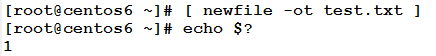• The following test checks whether bin is a directory.As bin is truly a directory, the exit status indicates the test evaluates to true.

• This test checks if there is file (of any type) with name foo.txt in the current directory.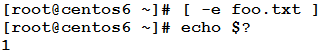• The following two test statements check if bin and newfile are ordinary files. As bin is a directory, the result of the first check is false (exit status 1). On the other hand, the second check evaluates to true because newfile is an ordinary file.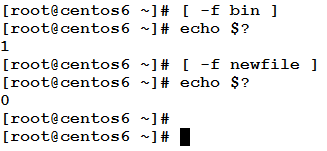• The following test statements check two files if their sizes are greater than zero. One file has non-zero size, which makes the test evaluates to true, while the other file emptyfile has zero size, which makes the test evaluates to false.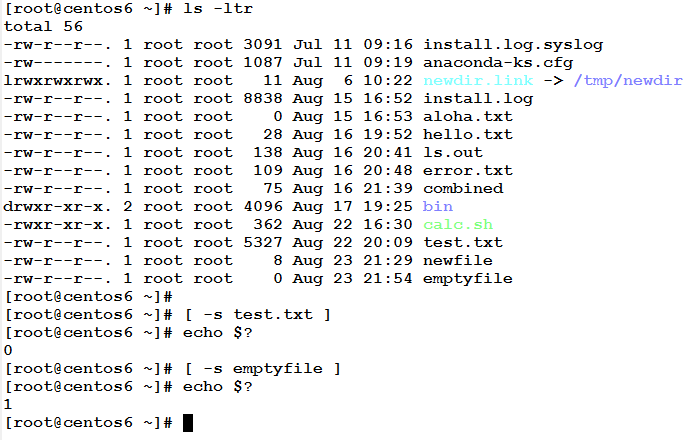Examples
The following examples illustrate how Logical Operators work.

Continuing the previous examples using File test operators, we need to check if a certain file exists, and it is an ordinary file, and its size is greater than 0. How could we achieve this?!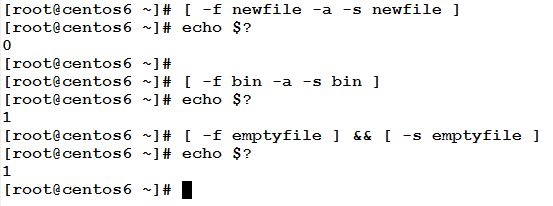The three test statements above uses the Logical AND operation to combine two test conditions: whether the file exits and it is a regular file –f , and whether its size is greater than zero.

The first condition succeeds because newfile is a regular file and its size is greater than zero. While the other two conditions fails for two different reasons: one because its type is a directory (not a regular file), and the other because its size is 0.

Now, we need to test for the existence of another file. The test should be true if the file exists and its type either an ordinary file or symbolic link.

Check this: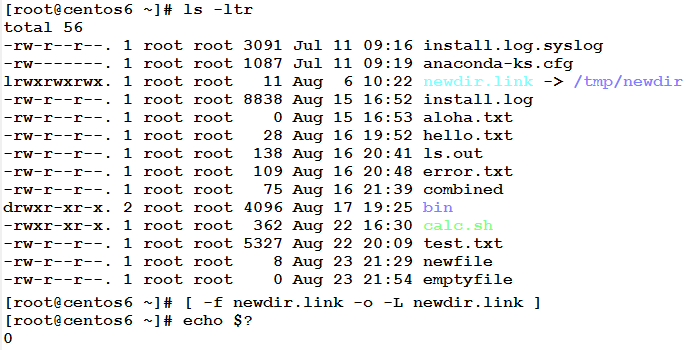The newdir.link is a symbolic link. When tested using the above composite condition, the first condition fails, but the second one succeeds, so the result of ORing the false and the true values becomes true (exit status 0).

* * * * * *

In this Article, we have talked about important topic. Understanding test conditions is necessary base for the upcoming topics: Decision making, and some types of Loops. So, if you miss something, kindly re-read the topic once again to make sure everything is clear.

See you in the next topic.

### Build A Front End Of an e-Commerce Site Using React

21,501Fans
4,213Followers
104,000Subscribers

### Build A Front End Of an e-Commerce Site Using React

- Advertisement -